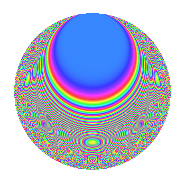# Properties

 Label 27.5.fLevel 27 Weight 5 Character orbit f Rep. character $$\chi_{27}(2,\cdot)$$ Character field $$\Q(\zeta_{18})$$ Dimension 66 Newform subspaces 1 Sturm bound 15 Trace bound 0

# Related objects

## Defining parameters

 Level: $$N$$ $$=$$ $$27 = 3^{3}$$ Weight: $$k$$ $$=$$ $$5$$ Character orbit: $$[\chi]$$ $$=$$ 27.f (of order $$18$$ and degree $$6$$) Character conductor: $$\operatorname{cond}(\chi)$$ $$=$$ $$27$$ Character field: $$\Q(\zeta_{18})$$ Newform subspaces: $$1$$ Sturm bound: $$15$$ Trace bound: $$0$$

## Dimensions

The following table gives the dimensions of various subspaces of $$M_{5}(27, [\chi])$$.

Total New Old
Modular forms 78 78 0
Cusp forms 66 66 0
Eisenstein series 12 12 0

## Trace form

 $$66q - 6q^{2} - 6q^{3} - 6q^{4} + 3q^{5} + 90q^{6} - 6q^{7} - 9q^{8} - 108q^{9} + O(q^{10})$$ $$66q - 6q^{2} - 6q^{3} - 6q^{4} + 3q^{5} + 90q^{6} - 6q^{7} - 9q^{8} - 108q^{9} - 3q^{10} - 492q^{11} - 339q^{12} - 6q^{13} + 1137q^{14} + 1017q^{15} - 54q^{16} - 9q^{17} + 603q^{18} - 3q^{19} - 2487q^{20} - 2784q^{21} + 1002q^{22} - 2724q^{23} - 3312q^{24} + 435q^{25} + 1368q^{27} - 12q^{28} + 5016q^{29} + 10773q^{30} - 1671q^{31} + 10224q^{32} + 2925q^{33} - 342q^{34} - 2682q^{35} - 7704q^{36} - 3q^{37} - 6564q^{38} - 1983q^{39} - 1113q^{40} + 5754q^{41} + 8694q^{42} - 1266q^{43} - 4545q^{44} - 7029q^{45} - 3q^{46} - 9159q^{47} - 28383q^{48} + 5898q^{49} - 34977q^{50} - 14814q^{51} + 2871q^{52} + 13878q^{54} - 12q^{55} + 39243q^{56} + 15792q^{57} - 12291q^{58} + 24762q^{59} + 50670q^{60} - 8358q^{61} + 38304q^{62} + 21609q^{63} + 6141q^{64} + 23727q^{65} + 11349q^{66} + 15996q^{67} - 43533q^{68} - 26847q^{69} - 7251q^{70} - 19773q^{71} - 77580q^{72} + 6108q^{73} - 74847q^{74} - 49155q^{75} - 28614q^{76} - 33909q^{77} - 9108q^{78} - 5658q^{79} - 12600q^{81} - 12q^{82} + 18813q^{83} + 82356q^{84} + 24219q^{85} + 96474q^{86} + 84555q^{87} + 36042q^{88} + 110232q^{89} + 153540q^{90} - 6042q^{91} + 73545q^{92} + 1293q^{93} + 2631q^{94} - 65163q^{95} - 152226q^{96} - 3696q^{97} - 259938q^{98} - 162405q^{99} + O(q^{100})$$

## Decomposition of $$S_{5}^{\mathrm{new}}(27, [\chi])$$ into newform subspaces

Label Dim. $$A$$ Field CM Traces $q$-expansion
$$a_2$$ $$a_3$$ $$a_5$$ $$a_7$$
27.5.f.a $$66$$ $$2.791$$ None $$-6$$ $$-6$$ $$3$$ $$-6$$

## Hecke characteristic polynomials

There are no characteristic polynomials of Hecke operators in the database